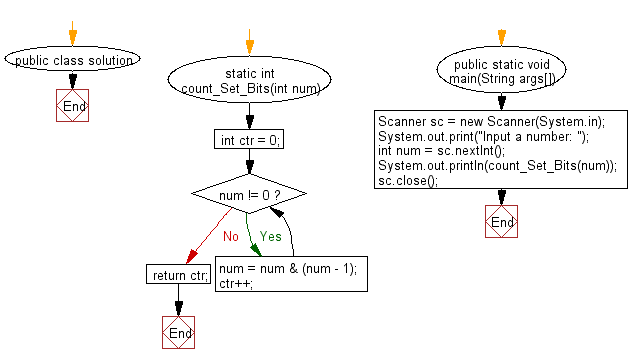# Java Exercises: Count the number of set bits in a 32-bit integer

## Java Basic: Exercise-249 with Solution

From Wikipedia,
The Hamming weight of a string is the number of symbols that are different from the zero-symbol of the alphabet used. It is thus equivalent to the Hamming distance from the all-zero string of the same length. For the most typical case, a string of bits, this is the number of 1's in the string, or the digit sum of the binary representation of a given number and the ℓ norm of a bit vector. In this binary case, it is also called the population count, popcount, sideways sum, or bit summation.

Example:

String Hamming weight
11101 4
11101000 4
00000000 0
789012340567 10
Write a Java program to count the number of set bits in a 32-bit integer.

Sample Solution:

Java Code:

``````import java.util.Scanner;

public class solution {

static int count_Set_Bits(int num) {
int ctr = 0;
while (num != 0) {
num = num & (num - 1);
ctr++;
}
return ctr;
}
public static void main(String args[]) {
Scanner sc = new Scanner(System.in);
System.out.print("Input a number: ");
int num = sc.nextInt();
System.out.println(count_Set_Bits(num));
sc.close();
}
}
```
```

Sample Output:

```Input a number:  1427
6
```

Flowchart:Java Code Editor:

What is the difficulty level of this exercise?

﻿

## Java: Tips of the Day

Array vs ArrayLists:

The main difference between these two is that an Array is of fixed size so once you have created an Array you cannot change it but the ArrayList is not of fixed size. You can create instances of ArrayLists without specifying its size. So if you create such instances of an ArrayList without specifying its size Java will create an instance of an ArrayList of default size.

Once an ArrayList is full it re-sizes itself. In fact, an ArrayList is internally supported by an array. So when an ArrayList is resized it will slow down its performance a bit as the contents of the old Array must be copied to a new Array.

At the same time, it's compulsory to specify the size of an Array directly or indirectly while creating it. And also Arrays can store both primitives and objects while ArrayLists only can store objects.

Ref: https://bit.ly/3o8L2KH